﻿ Clemson Vehicular Electronics Laboratory: The Method of Moments# The Method of Moments

In the 1960s, R.F. Harrington  and others applied a technique called the Method of Moments to the solution of electromagnetic field problems. The Method of Moments (also called the Method of Weighted Residuals) is a technique for solving linear equations of the form,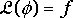(1)

where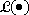is a linear operator, f is a known excitation or forcing function, and φ is an unknown quantity. To solve this problem on a digital computer, we start by expressing the unknown solution as a series of basis or expansion functions, vn,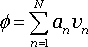(2)

where an are unknown coefficients describing the amplitude of each term in the series.

Now instead of one equation with a continuous unknown quantity, f, we have an equation with N scalar unknowns,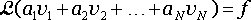(3)

To solve for the values of an, we need N linearly independent equations; so N different weighting or testing functions, wn, are applied. This yields the following system of N equations in N unknowns: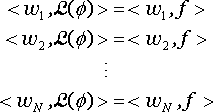(4)

Or, expressed in matrix form,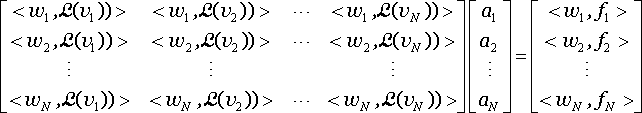(5)

This linear system of equations has the form,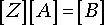(6)

where the elements of [Z] are known quantities that can be calculated from the linear operator,, and the chosen basis and weighting functions. The elements of [B] are determined by applying the weighting functions to the known forcing function. The unknown elements of [A] can be found by solving the matrix equation. After solving for [A] (i.e. the unknown coefficients, an), the value of φ is determined using Equation (2).

The Method of Moments (MoM) can be used to solve a wide range of equations involving linear operations including integral and differential equations. This numerical technique has many applications other than electromagnetic modeling; however the MoM is widely used to solve equations derived from Maxwell's equations. In general, moment method codes generate and solve large, dense matrix equations and most of the computational resources required are devoted to filling and solving this matrix equation. The particular form of the equations that is solved and the choice of basis and weighting functions have a great impact on the size of this matrix and ultimately the suitability of a given moment method code to model a given geometry.

## Equation Options

The most common equation form solved by CEM modeling codes based on the Method of Moments is the Electric Field Integral Equation (EFIE). This is an equation of the form,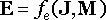(7)

where E is the impressed (i.e. source) electric field and J and M are the induced electric and magnetic current densities, respectively. The EFIE will be discussed further in the section describing the Boundary Element Method. Generally, codes that solve a form of the EFIE excel at modeling open (unbounded) geometries in which the electric field dominates in the near-field region of the source.

Another equation solved by Moment Method codes is the Magnetic Field Integral Equation (MFIE), which has the general form,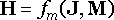(8)

where H is the impressed (i.e. source) magnetic field intensity. Codes that solve a form of the MFIE are best suited for modeling geometries with circulating currents, where the magnetic near field is dominant.

Moment Method codes based on the EFIE or MFIE alone, may exhibit unstable behavior when the modeling surfaces form a resonant cavity at a particular frequency. To avoid this, many moment method codes solve a linear combination of the EFIE and MFIE known as a Combined Field Integral Equation (CFIE). This requires more calculations to fill the matrix, but results in a more stable solution when the modeling surface is large enough to support an interior resonance.

Some CEM modeling codes employ the Method of Moments to solve other equations. For example, static modeling codes often solve a form of Laplace's equation relating electric field strengths to charge densities or magnetic field strengths to current densities. The Generalized Multiple Technique (GMT), which is described in another section of this report, employs a moment method to solve equations for the electric field generated by multipole sources.

## Basis and Weighting Functions

An appropriate choice of basis and weighting functions can make a tremendous difference in the number of elements, N, required to obtain an accurate solution. Since the solution is represented as a summation of basis functions [see Eq. (2)], it is important to choose basis functions that accurately represent the solution with a small number of terms. For example, when solving for the current distribution on a surface, the basis functions should be current distribution elements that can be summed together in a way that is able to efficiently approximate any overall current distribution that might result from the analysis.

Weighting functions should be chosen that maximize the linear independence of the various weighted forms of the equation. Often, the best choices of weighting functions are functions that are identical to the basis functions. Moment method techniques that employ identical basis and weighting functions are called Galerkin techniques.

## References

       R. F. Harrington, Field Computation by Moment Methods, Macmillan Publishing Company, New York, 1968. (reprinted by IEEE Press in 1993.)

       R. F. Harrington, Time Harmonic Electromagnetic Fields, New York, McGraw Hill, 1961, Ch. 3.

       R. E. Collin, Field Theory of Guided Waves, 2nd ed., New York, IEEE Press, 1991, Ch. 2.